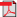# Search Results

1. E. Hyytiä, Dynamic Control of All-Optical WDM Networks, Licentiate thesis, Helsinki University of Technology, Networking Laboratory, 2001(bib)
Abstract: In this work the dynamic routing and wavelength assignment problem (D-RWA) in all-optical WDM network is studied. The problem is approached in the framework of the Markov Decision Process theory. In practice the optimal policy cannot be exactly calculated due to the huge size of the state space, but heuristic algorithms can come quite close to the optimal policy. The main contribution of the work is the application of so called first policy iteration to D-RWA. In the first policy iteration one tries to improve a given policy. This is done by considering the expected future costs that consist of the immediate cost of the chosen action and the relative cost of the next state. Immediate costs are known while the relative costs are not. Furthermore, the relative costs of the states cannot be obtained for every state due to the astronomical state space size. However, at each decision epoch the relative values are only needed for a small set of possible actions. Thus, instead of trying to solve them exactly their values are estimated by simulations. The work consists of four parts. In first part a brief introduction to all-optical WDM networks is given. The second part is a survey of the static routing and wavelength assignment problem, where the problem is described together with some heuristic RWA algorithms. The third part of the thesis considers the dynamic routing and wavelength assignment problem and contains, among other things, a description how the first policy iteration can be applied to D-RWA problem, together with some simulation results. The fourth part contains a brief survey of the important restoration and protection aspects in all-optical networks.